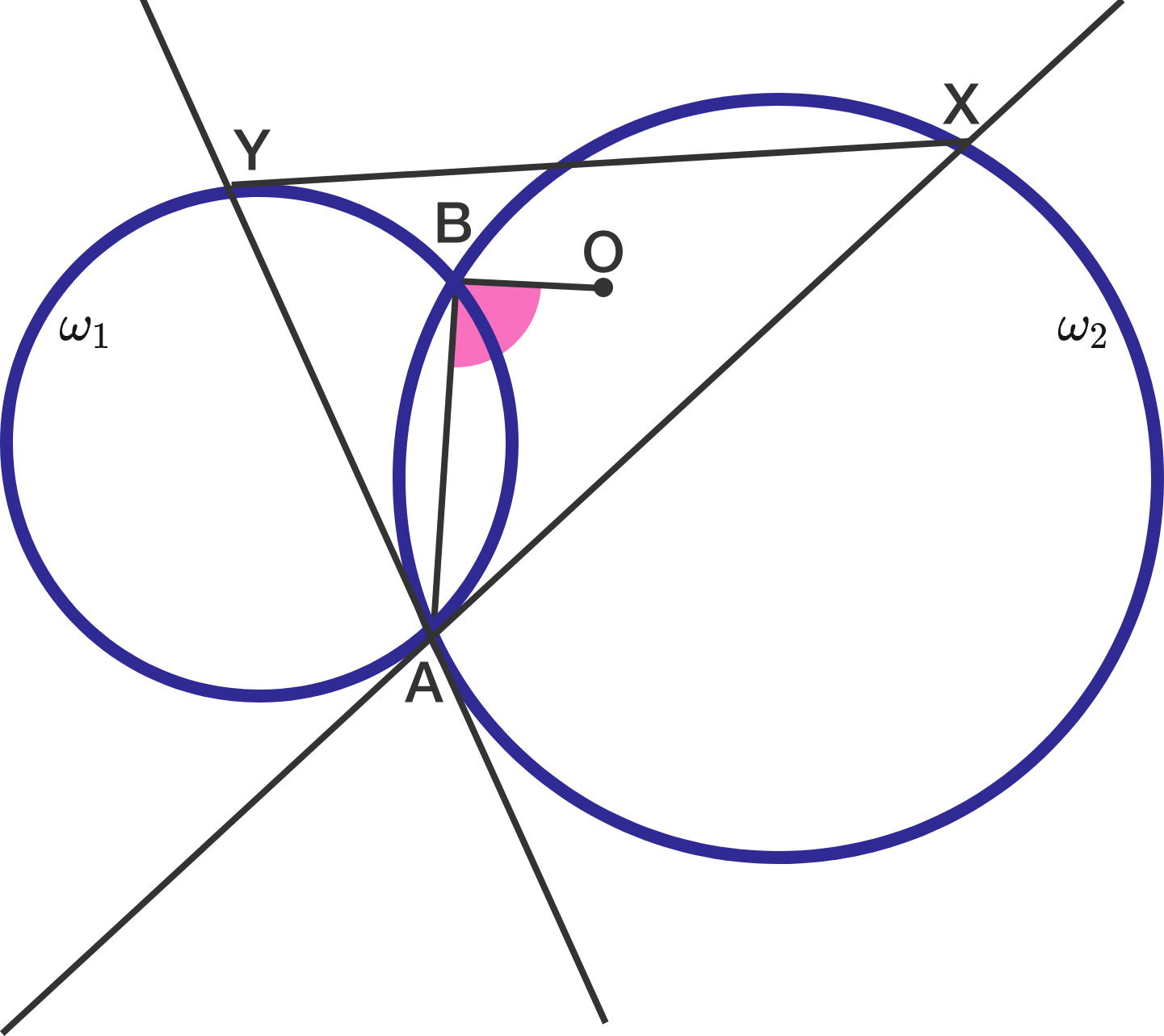# The Circumcircle And The TangentsTwo circles $\omega_1$ and $\omega_2$ intersect at points $A$ and $B$. The tangent to $\omega_1$ passing through $A$ intersects $\omega_2$ at $X$. The tangent to $\omega_2$ passing through $A$ intersects $\omega_1$ at $Y$. Let $O$ be the circumcenter of $\triangle XAY$. Then what is the measure of $\angle OBA$ in degrees?

×